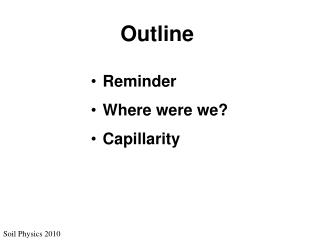DownloadDownload PresentationSoil Physics 2010

# Soil Physics 2010

Télécharger la présentation## Soil Physics 2010

- - - - - - - - - - - - - - - - - - - - - - - - - - - E N D - - - - - - - - - - - - - - - - - - - - - - - - - - -
##### Presentation Transcript

1. Outline • Reminder • Where were we? • Capillarity Soil Physics 2010

2. Reminder Homework 1 is due Soil Physics 2010

3. Uh-oh Quiz Soil Physics 2010

4. Where were we? Solutes lower the water’s energy Fresh water Salt water Water moves from higher (pure) to lower (salty) energy state Soil Physics 2010

5. How do we know it’s energy? At equilibrium, the higher pressure balances the energy-lowering effect of the salt. Dh Fresh water Salt water This is the osmotic pressure, P Soil Physics 2010

6. Water and heat Heat capacity in J/kg, liquid: Methane 4022 Ammonia 4708 Water 4186 HF 2580 Neon 2009 H2S 2509 Water is resistant to temperature change, including phase change Soil Physics 2010

7. Capillarity Soil Physics 2010 demoroom.physics.ncsu.edu

8. Cohesion Soil Physics 2010

9. Cohesion If each water molecule has the same cohesion, then molecules on an interface cohere preferentially inward and along the surface. Soil Physics 2010

10. A soap boat A floating body (length 2.5cm) contains a small volume of soap, which serves as its fuel in propelling it across the free surface. The soap exits the rear of the boat, decreasing the local surface tension. The resulting fore-to-aft surface tension gradient propels the boat forward. The water surface is covered with Thymol blue, which parts owing to the presence of soap, visible as a whitish streak. Soil Physics 2010 From John Bush, MIT

11. Surface tension Interfacial tension Surface energy One effect of surface energy is to minimize the surface area Soil Physics 2010

12. Surface tension One effect of surface energy is to minimize the surface area Soil Physics 2010

13. Adhesion But also: Water “sticks” to other materials, not just to itself Soil Physics 2010

14. Capillarity Bloody2.com demoroom.physics.ncsu.edu Soil Physics 2010

15. Capillarity At equilibrium, Force up = Force down Force = Mass * acceleration (Newton’s 2nd law) r Force down = mass * g = rw (p r2 h) * g (or better) = (rw – ra) * (p r2 h) * g h Soil Physics 2010

16. Capillarity At equilibrium, Force up = Force down • Force up is: • proportional to surface tension g • exerted around the inside perimeter of the tube r Force up = g * 2 p r Soil Physics 2010

17. Capillary rise equation Force down = (rw – ra) * (p r2 h) * g Force up = g * 2 p r At equilibrium, Force up = Force down r (rw – ra) * (p r2 h) * g = g * 2 p r h Soil Physics 2010

18. Wetting angle www.alsapaint.com Soil Physics 2010

19. Changing the wetting angle “A raindrop is extended like a film by water processing on the surface of a mirror, and the visibility in case of rainy weather is raised.” Honda ad, honda-car.jp www.croda.com Soil Physics 2010